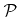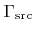Next: 5.2.4 Algorithmic Complexity Up: 5.2 Ray Tracing Previous: 5.2.2 Tangential Disks

## 5.2.3 Particle Traversal

Particles are consecutively launched from the source planeaccording to the arriving flux distribution. The vertical position ofcan be arbitrarily chosen, however, it must be above the surface. If the arriving flux distributionvaries spatially, it must be adapted correspondingly when shifting the source plane. For ray tracing a good choice is the lowest grid plane which is entirely above the surface. Hence, it is the first grid plane, where all grid points have a positive signed LS value. This plane limits the simulation domain on top. The simulation domain can also be limited at the bottom by the first grid plane which is entirely below the surface. Together with the lateral boundaries a rectangular simulation domain is obtained.

To find the first intersection of a particle trajectory with the surface all traversed grid cells need to be tested for an intersection within their interiors. If a surface intersection is found, new particles must be launched from the intersection point according to the reemission law. Their reemitted direction and energy as well as their particle species and their starting point are stored in a stack, because these particles will be processed later. First the trajectory of the incident particle hitting the surface is further calculated for a few grid spacings. This allows the proper calculation of the surface rates, even if the corresponding tangential disks are behind the surface (compare Figure 5.1). In this work the trajectories are prolonged for three grid spacings before discarding the particle and calculating the trajectory of the next one. The new particle is either obtained from the stack, if it is not empty, or else by generating a new particle launched from the source plane.

Continuous tests of all grid cell corners to check for active grid points, followed by the corresponding tangential disk intersection tests, lead to unnecessary computational effort. The same grid point will likely be tested multiple times within different neighboring grid cells. A better strategy is to test only those grid points opposite to the face, through which the particle enters the grid cell (see Figure 5.1). In this way each grid point is only tested once.Next: 5.2.4 Algorithmic Complexity Up: 5.2 Ray Tracing Previous: 5.2.2 Tangential Disks

Otmar Ertl: Numerical Methods for Topography Simulation